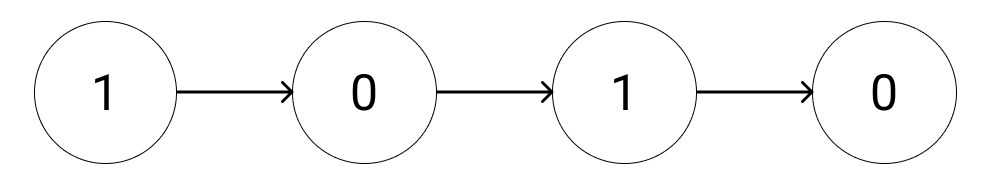New update is available. Click here to update.

# Binary Linked List To Integer

Last Updated: 22 Sep, 2021
Difficulty: Easy

## PROBLEM STATEMENT

#### You are given a singly linked list containing ‘n’ nodes, where every node in the linked list contains a pointer “next” which points to the next node in the list and having values either 0 or 1. Your task is to return the decimal representation of the given number in the linked list.

##### For Example:
``````n = 4, list: 1 -> 0 -> 1 -> 0.
````````````Now in this example, the value in the linked list is 1010, which is 10 in Decimal.
``````
##### Input Format :
``````The first line contains a single integer ‘T’ denoting the number of test cases. Then each test case follows:

The first line of each test case contains an integer, ‘n’, denoting the number of nodes in the linked list.

The next ‘M’ lines of the test case contain two space-separated integers, ‘x’ and ‘y’, denoting a track between ‘x’ and ‘y’.

The last line of the test case contains ‘n + 1’ space-separated integers which are the nodes of the linked list and each line ends with -1 to indicate that the sublist is over. Thus, -1 will never be a linked list element.
``````
##### Output Format :
``````For each test case, print the Decimal representation of the number.

Output for each test case will be printed in a separate line.
``````
##### Note :
``````You are not required to print anything; it has already been taken care of. Just implement the function and return the answer.
``````
##### Constraints :
``````1 <= T <= 100
1 <= N <= 3000

Time Limit: 1sec
``````## Approach 1

The approach is simple, you have to take an integer to store the final answer, and iterate through the list and add the current element to “ans” and multiply ans by 2.

The steps are as follows:

• Take an integer “num” and initialize it with zero.
• Iterate through the list till we reach the last element:
• Multiply “num” by 2.
• Update “num = “head” -> data.